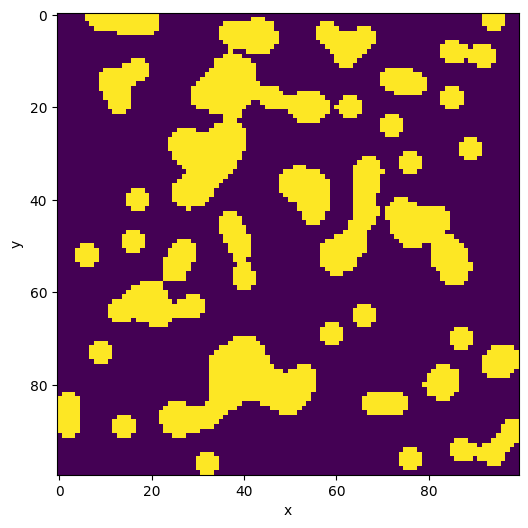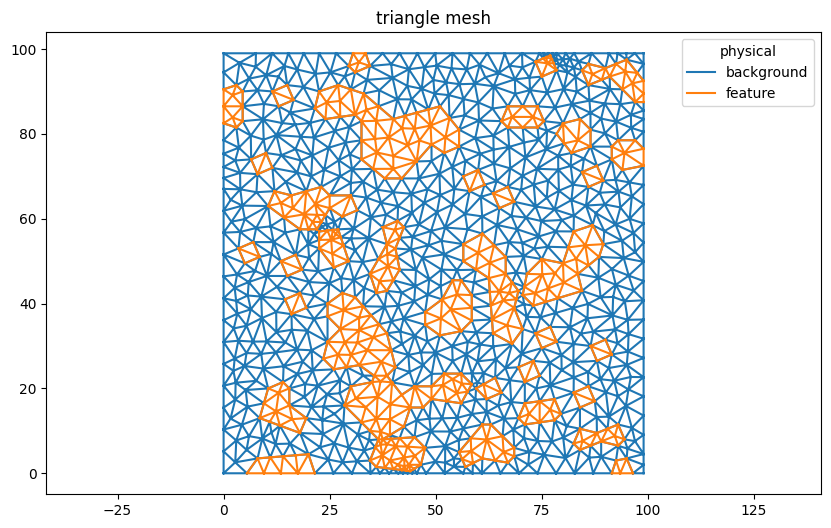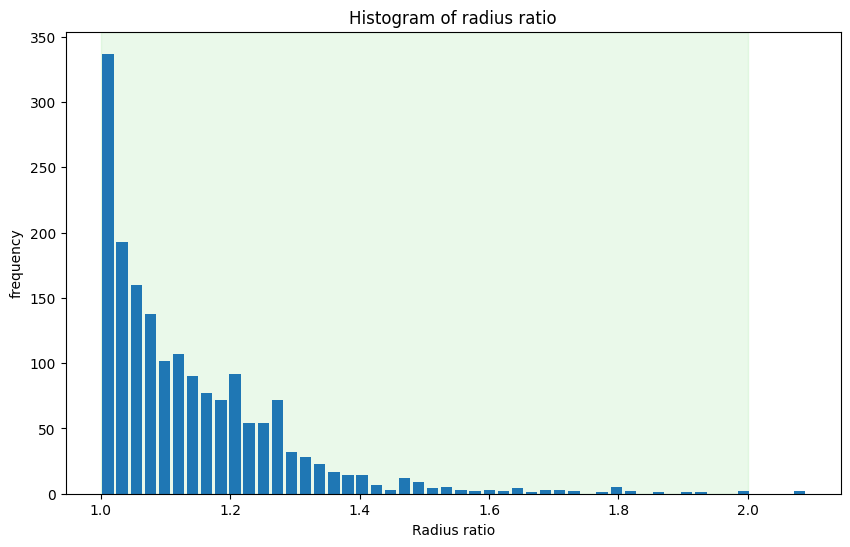:

%config InlineBackend.rc = {'figure.figsize': (10,6)}
%matplotlib inline


# Hello world!

This example shows the workflow for generating a mesh from segmented data, and demonstrates a few of the features of Nanomesh. It uses a synthetic binary image with several rounded blob-like objects generated by skimage.

Image data in Nanomesh is stored as an nanomesh.Image type. Calling nanomesh.Image will create the appropriate subtype, nanomesh.Plane for 2D or nanomesh.Volume for 3D data.

:

from skimage.data import binary_blobs
from nanomesh import Image

blobs = binary_blobs(length=100, volume_fraction=0.25, seed=2102)
plane = Image(blobs)

print(plane)

Plane(shape=(100, 100), range=(False,True), dtype=bool)


nanomesh.Image is essentially a container for a numpy array with some methods for image segmentation and visualization.

:

plane.show()

:

<AxesSubplot:xlabel='x', ylabel='y'>Generating a mesh from image data is simple in Nanomesh using nanomesh.Plane.generate_mesh(). The options opts are passed to the triangulation function (nanomesh.triangulate()). In this example, we use q30 to generate a quality mesh with minimum angles of 30°, and a50 to limit the triangle size to 50 pixels.

The returned mesh is a nanomesh.MeshContainer with the generated triangles and line segments.

:

mesh = plane.generate_mesh(opts='q30a10')
mesh

:

<MeshContainer>
Number of points: 932
Number of cells:
triangle: 1754
line: 2685
Point data: physical
Cell data: physical
Field data: feature, background


In the next cell, we plot the triangles.

:

mesh.plot('triangle')

:

<AxesSubplot:title={'center':'triangle mesh'}>Nanomesh can also calculate cell quality metrics and show them as a colored triangle or histogram plot.

Note that the area shaded in green below highlights the optimal range.

Have a look at the nanomesh.metrics submodule or in the example: Calculate mesh quality metrics.

:

from nanomesh import metrics

triangle_mesh = mesh.get('triangle')


:

<AxesSubplot:title={'center':'Histogram of radius ratio'}, xlabel='Radius ratio', ylabel='frequency'>Nanomesh uses [meshio](https://github.com/nschloe/meshio) to write data to most meshing formats.

:

mesh.write('mesh.vtk')

Warning: VTK requires 3D points, but 2D points given. Appending 0 third component.


For more practical examples of how to use Nanomesh, check out the how-to guides and notebooks: Overview.

Generated by nbsphinx from a Jupyter notebook.